Courses

# NCERT Solutions(Part - 1) - Exponents and Powers Class 7 Notes | EduRev

## Class 7 : NCERT Solutions(Part - 1) - Exponents and Powers Class 7 Notes | EduRev

The document NCERT Solutions(Part - 1) - Exponents and Powers Class 7 Notes | EduRev is a part of the Class 7 Course Mathematics (Maths) Class 7.
All you need of Class 7 at this link: Class 7

Exercise 13.1

Question 1: Find the value of:

(i) 2

(ii) 9

(iii) 11

(iv) 5

(i) 26 =2x2x2x2x2x2 = 64
(ii) 93 = 9x9x9 = 729
(iii) 11= 11 x 11= 121
(iv) 54 =5 x 5 x 5 x 5 = 625

Question 2: Express the following in exponential form:

(i) 6 x 6 x 6 x 6
(ii) t x t
(iii) b x b x b x b
(iv) 5 x 5 x 7 x 7 x 7
(v) 2 x 2 x a x a
(vi) a x a x a x c x c x c x c x d

(i) 6 x 6 x 6 x 6 = 64
(ii) t x t = t2
(iii) b x b x b x b = b4
(iv) 5 x 5 x 7 x 7 x 7 = 52 x 73
(v) 2 x 2 x a x a = 2x a2
(vi) a x a x a x c x c x c x c x d = a3 x c4 x d

Question 3: Express each of the following numbers using exponential notation:

(i) 512
(ii) 343
(iii) 729
(iv) 3125

(i) 512

512 = 2 x 2 x 2 x 2 x 2 x 2 x 2 x 2 x 2 = 29

 2       512 2 256 2 128 2 64 2 32 2 16 2 8 2 4 2 2 1

(ii) 343
343 = 7 x 7 x 7 = 73

 7       343 7 49 7 7 1

(iii) 729
729 = 3 x 3 x 3 x 3 x 3 x 3 = 36

 3       729 3 243 3 81 3 27 3 9 3 3 1

(iv) 3125
3125 = 5 x 5 x 5 x 5 x 5

 5 3125 5 625 5 125 5 25 5 5 1

Question 4: Identify the greater number, wherever possible, in each of the following:

(i)  43 and 34
(ii)  53 or 35
(iii) 28 or 82
(iv) 100or 2100
(v) 210 or 102

(i) 43 = 4 x 4 x 4 = 64
34 = 3 x 3 x 3 x 3 = 81
Since 64 < 81
Thus, 34 is greater than 43.

(ii) 53 = 5 x 5 x 5 = 125
35 = 3 x 3 x 3 x 3 x 3 = 243
Since, 125 < 243
Thus, 34 is greater than 53.

(iii) 28= 2 x 2 x 2 x 2 x 2 x 2 x 2 x 2 = 256
82 = 8x8 = 64
Since, 256 > 64
Thus, 28 is greater than 82.

(iv) 1002 = 100 x 100 = 10,000
2100 = 2 x 2 x 2 x 2 x 2 x.......14 times x....x 2 = 16,384 x....x 2
Since, 10,000 < 16,384 x....x2
Thus, 2100 is greater than 1002.

(v)  210 = 2 x 2 x 2 x 2 x 2 x 2 x 2 x 2 x 2 x 2 = 1,024
102 = 10x10 = 100
Since, 1,024 > 100
Thus, 210 >102

Question 5: Express each of the following as product of powers of their prime factors:

(i) 648

(ii) 405

(iii) 540

(iv) 3,600

(i)    648 = 23 x 34

 2 648 2 324 2 162 3 81 3 27 3 9 3 3 1

(ii) 405 = 5 x 34

 5 405 3 81 3 27 3 9 3 3 1

(iii) 540 = 22 x 33 x 5

 2 540 2 270 3 135 3 45 3 15 5 5 1

(iv) 3,600 = 24 x 3x 52

 2 3600 2 1800 2 900 2 450 3 225 3 75 5 25 5 5 1

Question 6: Simplify:

(i)  2 x 103
(ii) 72 x 22
(iii) 23 x 5
(iv) 3 x 44
(v) 0 x 102
(vi) 5x 33
(vii) 24 x 32
(viii) 32 x 104

(i)  2 x 103  = 2 x 10 x 10 x 10  = 2,000
(ii)  72 x 22 = 7 x 7 x 2 x 2  = 196
(iii) 23 x 5 = 2 x 2 x 2 x 5  = 40
(iv) 3 x 44 = 3 x 4 x 4 x 4 x 4  = 768
(v) 0 x 102 = 0 x 10 x 10  = 0
(vi) 53 x 33 = 5 x 5 x 3 x 3 x 3  = 675
(vii) 2x 32  = 2 x 2 x 2 x 2 x 3 x 3  = 144
(viii) 32 x 104 = 3 x 3x 10x10x 10x10  =  90,000

Question 7: Simplify:

(i) (-4)3
(ii) (-3)x(-2)3
(iii) (-3)x (-5)2
(iv) (-2)3 x (-10)3

(i)  (-4)3 = (-4)x(-4)x(-4) = -64
(ii) (-3)x(-2)3 =(-3)x(-2)x(-2)x(-2) = 24
(iii) (-3)2 x (-5)2 = (-3)x(-3)x(-5) x (-5) = 225
(iv) (-2)3x(-10)3 =(-2)x(-2)x(-2)x(-10)x(-10)x(-10)

Question 8: Compare the following numbers:

(i) 2.7 x 1012; 1.5 x 108

(ii) 4 x 1014; 3 x 1017

(i) 2.7 x 1012 and 1.5 x 108
On comparing the exponents of base 10,
2.7 x 1012 > 1.5x108

(ii) 4 x 1014 and 3 x 1017
On comparing the exponents of base 10,
4 x 1014 < 3 x 1017

Exercise 13.2

Question 1: Using laws of exponents, simplify and write the answer in exponential form:

(i) 32 x 34 x 38
(ii) 615 ÷ 610
(iii) a3 x a
(iv) 7x x 72
(v) (52)2 ÷ 53
(vi) 25 x 55
(vii) a4 x b4
(viii) (34)3
(ix) [220 ÷ 215) x 23

(x) 8t x 82

(i)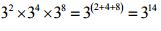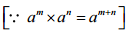(ii)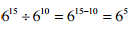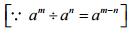(iii)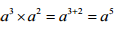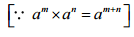(iv)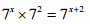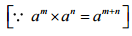(v)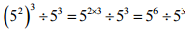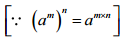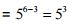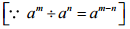(vi)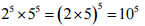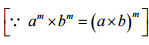(vii)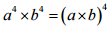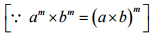(viii)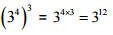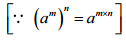(ix)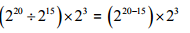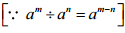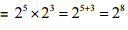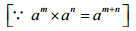(x)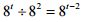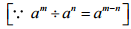Question 2: Simplify and express each of the following in exponential form:

(i)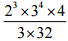(ii)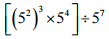(iii)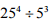(iv)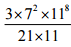(v)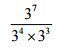(vi)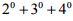(vii)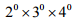(viii)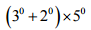(ix)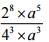(x)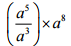(xi)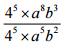(xii)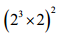(i)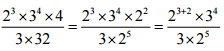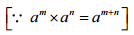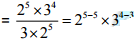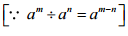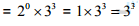(ii)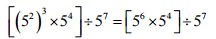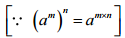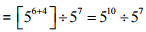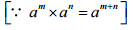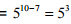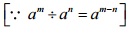(iii)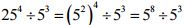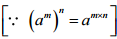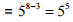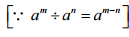(iv)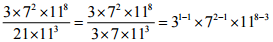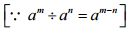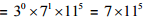(v)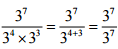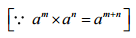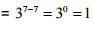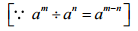(vi)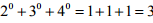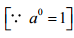(vii)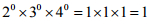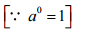(viii)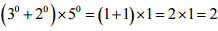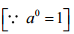(ix)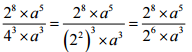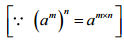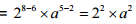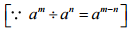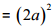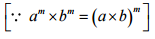(x)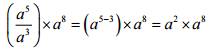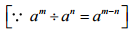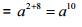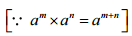(xi)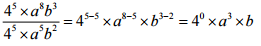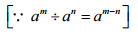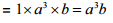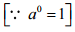(xii)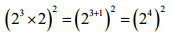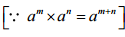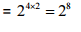(i) 10 x 1011 = 10011
(ii)  23 > 52
(iii) 23x 32 = 6s
(iv) 30 = (1000)0

(i) 10x1011 = 10011
L.H.S. 101+11 = 1012    and R.H.S. (102)11=1022
Since, L.H.S. ≠ R.H.S.
Therefore, it is false.

(ii) 23 > 52
L.H.S 23 = 8    and R.H.S. 52 = 25
Since, L.H,S. is not greater than R.H.S.
Therefore, it is false,

(iii) 23 x32 = 65
L.H.S. 23 x 32 = 8 x 9 = 72    and R.H.S. 65 =7.776
Since, L.H.S. ≠ R.H.S.
Therefore, it is false.

(iv) 3° = (1000)°
L.H.S. 3° = 1    and R.H.S. (1000)° = 1
Since, L.H.S. = R.H.S.
Therefore, it is true.

Question 4: Express each of the following as a product of prime factors only in exponential form:
(i) 108 x 192
(ii) 270
(iii) 729 x 64
(iv) 768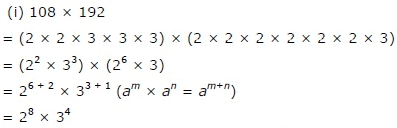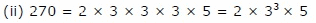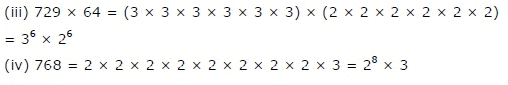Question 5: Simplify:

(i)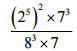(ii)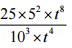(iii)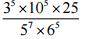(i)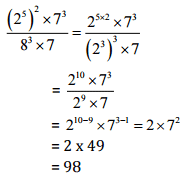(ii)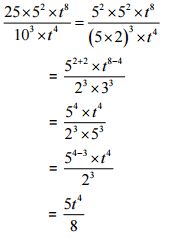(iii)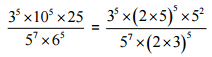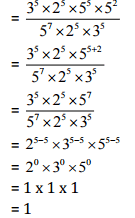Offer running on EduRev: Apply code STAYHOME200 to get INR 200 off on our premium plan EduRev Infinity!

## Mathematics (Maths) Class 7

161 videos|237 docs|45 tests

,

,

,

,

,

,

,

,

,

,

,

,

,

,

,

,

,

,

,

,

,

;